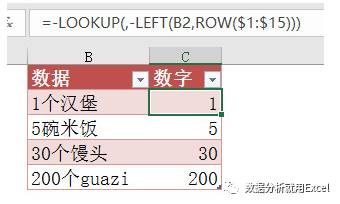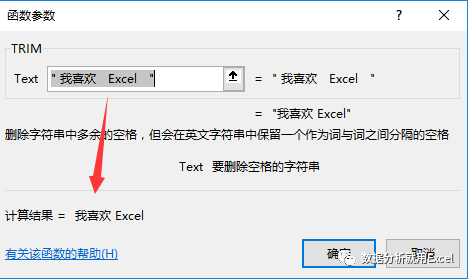# 【Excel函数贴】五个技巧性函数小套路

…………

1，

=UPPER("excel")

=LOWER("EXCEL")

=PROPER("excel")

2，

LOOKUP函数的四个经典套路。

=LOOKUP(9^9,A:A)

=LOOKUP("座",A:A)

=LOOKUP(1,0/(A:A<>""),A:A)

=LOOKUP(A1,{0,60,80,90},{"不及格","及格","良好","优秀"})

3，

=-LOOKUP(,-LEFT(B2,ROW(\$1:\$15)))4，

=TRIM(" 我喜欢  Excel   ")。5，

=IF(COUNTIF(D:E,A1),"存在","不存在")

=IF(COUNTIF(D:E,A1)>0,"存在","不存在")

COUNTIF的结果必然是一个大于0的数值。

COUNTIF结果必然为0。

0.1也是真的，哪怕是负数也是真的。